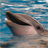# QlikView App Dev

Discussion Board for collaboration related to QlikView App Development.

Announcements
QlikWorld 2023, a live, in-person thrill ride. April 17 - 20, 2023, in Las Vegas! REGISTER TODAY
cancel
Showing results for
Did you mean:Contributor II

## Help in Expression

Hello Everyone,

I have bar chart with 4 expressions and one Drill down Dimension(Country-State-Account). I have field called 'Status '.Expressions I gave

sum((if([Status]='Open',1,0))) - for Open Account

'Percentage Open='&Num((sum((if([Status]='Active',1,0)))/(Count([Account]))),'#,##0.0%')

=sum((if([Case Status]='Closed',1,0)))- For Closed Account

'Percentage Closed='&Num((sum((if(Status]='Closed',1,0)))/(Count([Account]))),'#,##0.0%')

Though I gave these 4 expressions in bar chart when I drill down to Account level - it is showing canceled Accounts too..

My requirement is - it should not involve canceled accounts. How to modify the formula to achieve this. Please help me asap. Thank you!

1 Solution

Accepted SolutionsCreator

Please have a look into set analysis.

example to count or sum facts based on a preselected deminsion.

=sum({<Status = {'Open'}>}<amount>)

where <amount>  is the field you want to sum. you of course also could use a count.Creator

Please have a look into set analysis.

example to count or sum facts based on a preselected deminsion.

=sum({<Status = {'Open'}>}<amount>)

where <amount>  is the field you want to sum. you of course also could use a count.Community Browser